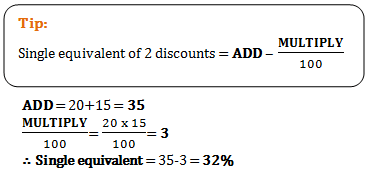# Aptitude test for Teachers - Set 2

1)   Three solid metallic balls of diameter 3cm, 4cm and 5m are melted into a solid sphere. The radius of new sphere is

a. 6 cm
b. 4 cm
c. 3 cm
d. 5 cm
 Answer  Explanation ANSWER: 3 cm Explanation: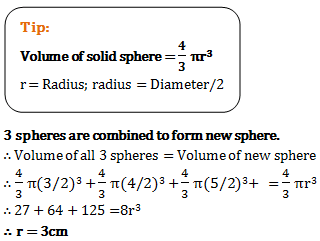2)   A boy was asked to multiply a number by 12. But he multiplied by 21 and got his answer 63 more than the correct answer. The number to be multiplied was

a. 7
b. 9
c. 8
d. 12
 Answer  Explanation ANSWER: 7 Explanation: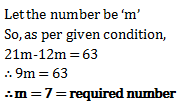3)   A number is multiplied by its one-third to get 192. Find the number.

a. 16
b. 20
c. 24
d. 28
 Answer  Explanation ANSWER: 24 Explanation: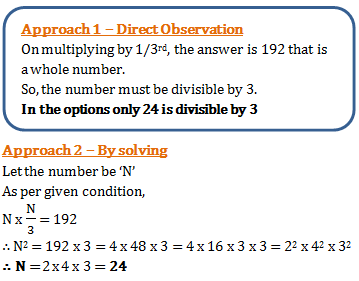4)   What is smallest number with which 5400 may be multiplied so that the product is perfect cube?

a. 5
b. 3
c. 4
d. 6
 Answer  Explanation ANSWER: 5 Explanation: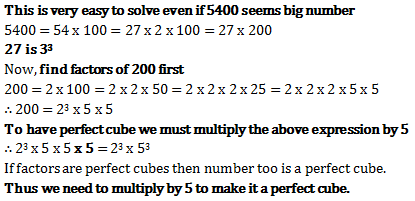5)   280 oranges are divided among some boys and girls whose total number is 50 so that each boy gets 5 oranges and each girl get 7 oranges. The number of girls is

a. 30
b. 35
c. 15
d. 20
 Answer  Explanation ANSWER: 15 Explanation: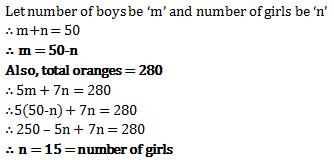6)   The sum of four consecutive integers is 1290. The greatest of them is?

a. 321
b. 322
c. 323
d. 324
 Answer  Explanation ANSWER: 324 Explanation: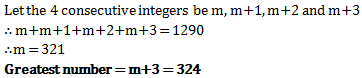7)   ¾ part of tank is full of water. When 30 litres of water is taken out it becomes empty. The capacity of the tank is?

a. 36 litres
b. 42 litres
c. 40 litres
d. 38 litres
 Answer  Explanation ANSWER: 40 litres Explanation: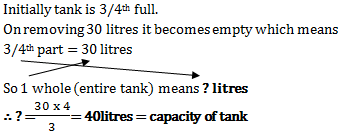8)   Two numbers are in ratio 2:3. If 2 be subtracted from the first and 2 be added to the second, the ratio becomes 1:2. Find the sum of the numbers.

a. 30
b. 28
c. 24
d. 10
 Answer  Explanation ANSWER: 30 Explanation: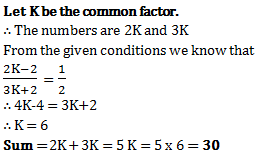9)   The greatest length of the scale that can measure exactly 30 cm, 90 cm, 1 m 20 cm and 1 m 35 cm lengths is

a. 5 cm
b. 10 cm
c. 15cm
d. 30 cm
 Answer  Explanation ANSWER: 15cm Explanation: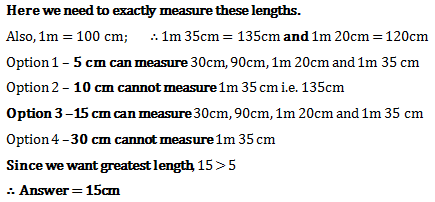10)   The value of 30.000027 x 0.008 is

a. 0.0006
b. 0.06
c. 0.006
d. 0.6
 Answer  Explanation ANSWER: 0.006 Explanation: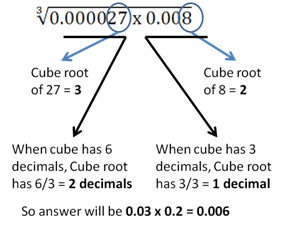11)   What is value of
 0.125 + 0.027 0.5 x 0.5 + 0.09 - 0.15

a. 1
b. 0.2
c. 0.08
d. 0.8
 Answer  Explanation ANSWER: 0.8 Explanation: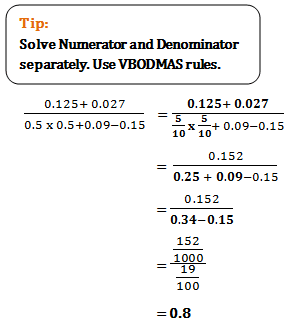12)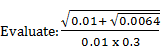a. 1
b. 10
c. 100
d. 1000
 Answer  Explanation ANSWER: 100 Explanation: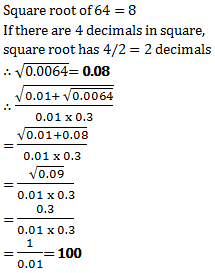13)   Express 2/3 of 1/4 of Rs.25.20 as a fraction of 1 1/2 of Rs. 36

a. 7/90
b. 11/90
c. 5/8
d. 5/42
 Answer  Explanation ANSWER: 7/90 Explanation: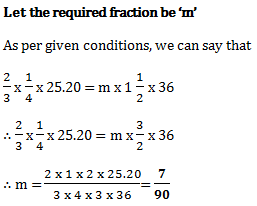14)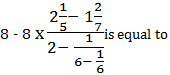a. 2
b. 4
c. 8
d. 6
 Answer  Explanation ANSWER: 4 Explanation: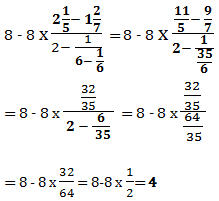15)   Which is largest amongst 5/8, 2/3, 7/9, 3/5?

a. 5/8
b. 2/3
c. 7/9
d. 3/5
 Answer  Explanation ANSWER: 7/9 Explanation: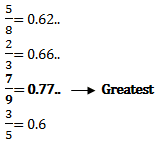16)   On simplification of the following, the result will be
(1 - 1/2)(1 - 1/3)(1 - 1/4)……..(1 - 1/100)

a. 0.01
b. 0.001
c. 1
d. 0.1
 Answer  Explanation ANSWER: 0.01 Explanation: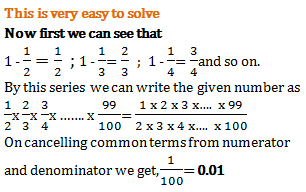17)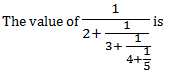a. 5/46
b. 68/157
c. 157/68
d. 21/110
 Answer  Explanation ANSWER: 68/157 Explanation: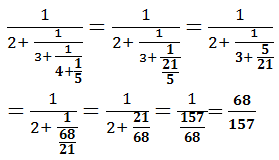18)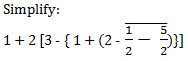a. 2
b. 1
c. -3
d. 5
 Answer  Explanation ANSWER: -3 Explanation: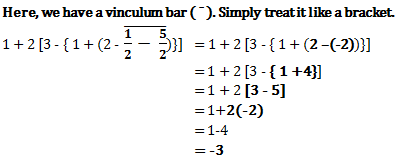19)   A shopkeeper earns a profit of 15% after selling a book at 20% discount on the printed price. The ratio of the cost price and printed price of the book is?

a. 20:23
b. 23:20
c. 16:23
d. 23:16
 Answer  Explanation ANSWER: 16:23 Explanation: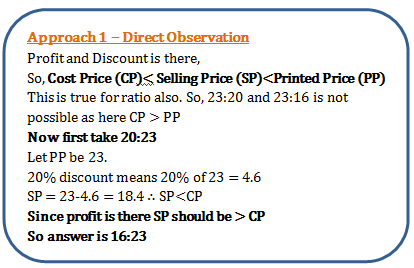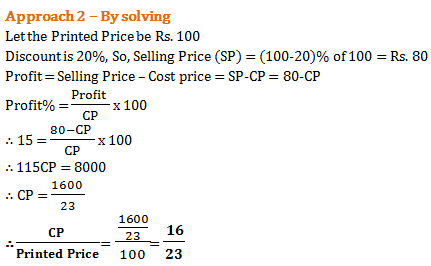20)   Successive discounts of 20% and 15% are equivalent to a single discount of

a. 68%
b. 65%
c. 35%
d. 32%
 Answer  Explanation ANSWER: 32% Explanation: